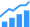Just because you migrate to the finFET process doesn’t mean you get the expected results.Power has been an important design challenge for quite some time. Leakage power started to grow in 90nm, and by 65nm it became a severe design issue.

We have built many techniques to address leakage, most notably power gating. These techniques are complex and have an impact on the design as a whole. FinFET technologies are seen as a boon to this issue of leakage. There are references that quote that leakage has been reduced by 60% compared to planar transistors. Does this mean that low power is not a focus anymore? Can we abandon all the low power techniques we have built over time?

Not so fast. To answer these questions, let’s look at an example design. Let’s assume that this is in some non-finFET process and has 1 unit of power in leakage. Assume that the dynamic power component is 9 units of power (90% dynamic and 10% leakage). Now assume that the chip is active during 10% of the time and in idle mode the remainder of the time. We can simply do the math and compute the total power as 1.8 units of power. Out of this, power is evenly split between active mode power and idle mode power (0.9 units each). This is the reason for employing leakage reduction techniques at this node.

Now, if the chip were designed in a finFET process, the leakage goes down by 60% to 0.3 units. Due to gate capacitance increase and the voltages not scaling with the process (refer), let us assume that the dynamic power goes up by 25%. Then the dynamic power is 11.25 units of power. If you assume that the activity profile of the chip has not changed, then the total power is about 1.48 units, which is 18% lower power compared to the non-finFET process. If we examine the individual components, the contribution of active power is 76% and the idle power contribution is only 24%. While the idle mode power has gone down considerably by 78% to just 0.36 units, dynamic power has increased by 52% to 1.13 power units.What are the conclusions from this analysis? Adopting finFET processes is an effective way to reducing power. But instead of throwing away all the low power techniques built from previous processes, the focus should be on mitigating dynamic power as it has become the dominant component of total power. Dynamic power reduction requires deeper understanding of the design and eliminating unnecessary activity that does not contribute to the functionality. Now, who in the project team is best capable of driving this activity?